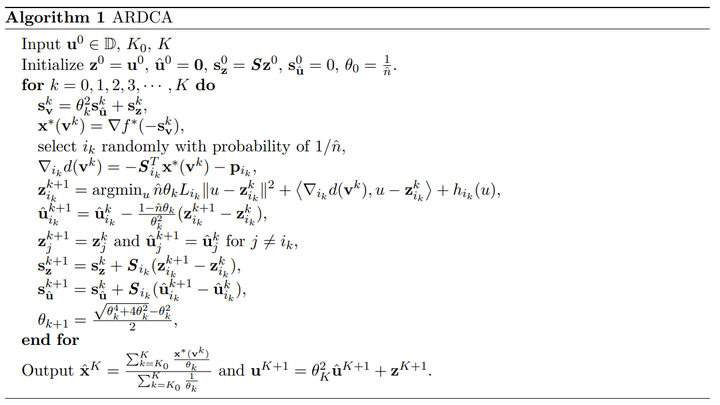# On the Complexity Analysis of the Primal Solutions for the Accelerated Randomized Dual Coordinate Ascent### Abstract

Dual first-order methods are essential techniques for large-scale constrained convex optimization. However, when recovering the primal solutions, we need $T(/epsilon^{-2})$ iterations to achieve an $/epsilon$-optimal primal solution when we apply an algorithm to the non-strongly convex dual problem with $T(/epsilon^{-1})$ iterations to achieve an $/epsilon$-optimal dual solution, where $T(x)$ can be $x$ or $/sqrt{x}$. In this paper, we prove the equal $O(/frac{1}{/sqrt{/epsilon}})$ iteration complexity of the primal solutions and dual solutions for the accelerated randomized dual coordinate ascent. When the dual function further satisfies the weak strong convexity condition, we establish the linear $O(/log/frac{1}{/epsilon})$ iteration complexity for both the primal solutions and dual solutions. When applied to the regularized empirical risk minimization problem, we prove the iteration complexity of $O(n/log n+/sqrt{/frac{n}{/epsilon}})$ in both primal space and dual space, where $n$ is the number of samples. Our result takes out the $(/log /frac{1}{/epsilon})$ factor compared with the methods based on smoothing/regularization or Catalyst reduction. As far as we know, this is the first time that the optimal $O(/sqrt{/frac{n}{/epsilon}})$ iteration complexity in the primal space is established for the dual coordinate ascent based stochastic algorithms. We also establish the accelerated linear complexity for some problems with nonsmooth loss, i.e., the least absolute deviation and SVM.

Type
Publication
Journal of Machine Learning Research